# ski-in / ski-out - Page 29

Viewing mode
697 results
• Slopes access :
• ski-in (-50 m)
• Rooms :
• Studio
• Total surface (sq.m) :
• 32 sq.m
•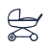•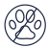• Slopes access :
• 50 -300m to the slopes
• Rooms :
• 2 rooms
• Total surface (sq.m) :
• 30 sq.m
••• Slopes access :
• 50 -300m to the slopes
• Rooms :
• 4 rooms
• Total surface (sq.m) :
• 85 sq.m
•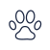•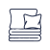•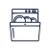• Slopes access :
• ski-in (-50 m)
• Total surface (sq.m) :
• 69 sq.m
••••• Slopes access :
• ski-in (-50 m)
• Rooms :
• Studio
• Studio + cabin(s)
• Total surface (sq.m) :
• 31 sq.m
•••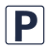•• Slopes access :
• 50 -300m to the slopes
• Rooms :
• Studio
• Studio + cabin(s)
• Total surface (sq.m) :
• 42 sq.m
•••• Slopes access :
• 50 -300m to the slopes
• Rooms :
• Studio
• Studio + cabin(s)
• Total surface (sq.m) :
• 30 sq.m
•••• Slopes access :
• ski-in (-50 m)
• Rooms :
• 2 rooms
• 2 rooms + cabin(s)
• Total surface (sq.m) :
• 50 sq.m
••••• Slopes access :
• 50 -300m to the slopes
• Rooms :
• 2 rooms
• Total surface (sq.m) :
• 40 sq.m
•••• Slopes access :
• ski-in (-50 m)
• Rooms :
• 2 rooms
• 2 rooms + cabin(s)
• Total surface (sq.m) :
• 25 sq.m
•••• Slopes access :
• 50 -300m to the slopes
• Rooms :
• 3 rooms
• Total surface (sq.m) :
• 44 sq.m
••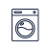••• Slopes access :
• 50 -300m to the slopes
• Rooms :
• 7 rooms
• Total surface (sq.m) :
• 170 sq.m
•••••• Slopes access :
• ski-in (-50 m)
• Rooms :
• 2 rooms
• 2 rooms + cabin(s)
• Total surface (sq.m) :
• 42 sq.m
••••• Slopes access :
• 50 -300m to the slopes
• Rooms :
• 2 rooms
•••• Slopes access :
• ski-in (-50 m)
• Rooms :
• Duplex
• Total surface (sq.m) :
• 50 sq.m
•••• Slopes access :
• 50 -300m to the slopes
• Rooms :
• 3 rooms
• Total surface (sq.m) :
• 80 sq.m
••••• Slopes access :
• ski-in (-50 m)
• Rooms :
• 3 rooms
• Total surface (sq.m) :
• 60 sq.m
•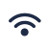• Garage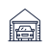•••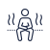•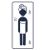•• Slopes access :
• ski-in (-50 m)
• Rooms :
• Studio
• Studio + cabin(s)
• Total surface (sq.m) :
• 30 sq.m
• Garage•••• Slopes access :
• ski-in (-50 m)
• Rooms :
• Studio + cabin(s)
• Total surface (sq.m) :
• 30 sq.m
••••• Slopes access :
• ski-in (-50 m)
• Rooms :
• Studio
• Total surface (sq.m) :
• 20 sq.m
•••